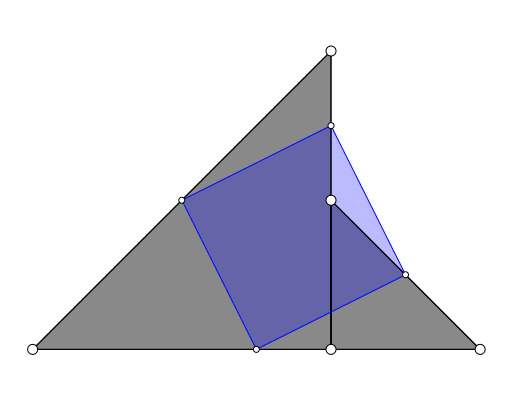# A geometry problem by Áron Bán-Szabó

Geometry Level 2

The two gray triangles are isosceles and right-angled. The midpoints of the quadrilateral defined by the triangles define the blue quadrilateral. Is the blue quadrilateral always a square?×